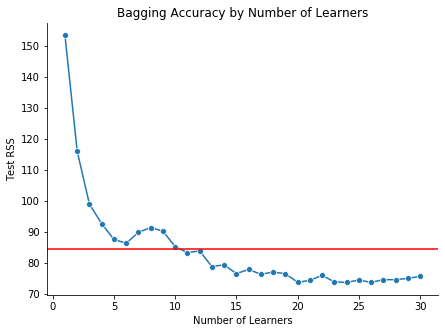# Concept¶

Due to their high variance, decision trees often fail to reach a level of precision comparable to other predictive algorithms. In the previous chapter, we introduced several ways to minimize the variance of a single decision tree, such as through pruning or direct size regulation. This chapter discusses another approach: ensemble methods. Ensemble methods combine the output of multiple simple models, often called “learners”, in order to create a final model with lower variance.

We will introduce ensemble methods in the context of tree-based learners, though ensemble methods can be applied to a wide range of learning algorithms. That said, the structure of decision trees makes ensemble methods particularly valuable. Here we discuss three tree-based ensemble methods: bagging, random forests, and boosting.

An example demonstrating the power of ensemble methods is given below. Using the tips dataset from scikit-learn, we build several tree-based learners. Then for $$b = 1, 2, \dots, 30$$, we use bagging to average the results of the $$b$$ learners. For each bagged model, we also calculate the out-of-sample $$RSS$$. The blue line below shows the $$RSS$$ for each bagged model—which clearly decreases with $$b$$—and the red line shows the $$RSS$$ for a single decision tree. By averaging many trees, rather than relying on a single one, we are able to improve the precision of our model.